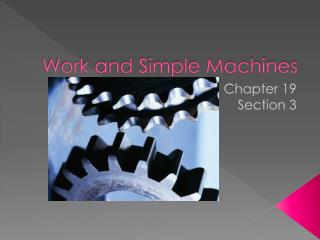DownloadDownload PresentationWork and Simple Machines

# Work and Simple Machines

Télécharger la présentation## Work and Simple Machines

- - - - - - - - - - - - - - - - - - - - - - - - - - - E N D - - - - - - - - - - - - - - - - - - - - - - - - - - -
##### Presentation Transcript

1. Work and Simple Machines Chapter 19 Section 3

2. Work • When a force causes an object to move in the same direction of the force that is being applied • Does effort always equal work? • Calculating Work • Work = force x distance or • W = F*d • Units as Force is measured in newtons (N) and distance in meters (m), thus work is measured in joules (J)

3. Calculating Work • A weight lifter lifts 500-N weight at distance of 2 m from the floor to a position over his head. How much work does he do? Where w = F*d

4. Calculating Work • Using a force of 50 N, you push a computer cart 10 m across a classroom floor. How much work did you do? • Work

5. Machine • What is a machine • A device that helps you do work • Simple machine • A machine that uses only one movement • Ex: Screwdriver, inclined plane, wedge, wheel, axel • Compound Machine • A combination of simple machines • Ex: can opener • How do machines make things easier • Increase the amount of force • Can change the direction of the force

6. Mechanical Advantage • The number of times the applied force is increased by a machine • Mechanical advantage = output force/input force • Or MA = Fo/Fi • Work In and Work Out • In simple machines the input and output force do work • Ex: Can opener the output force is at the blade puncturing the can • Ideal world there is no friction and work in = work out

7. The Pulley • Pulley – an object with a groove, like a wheel, with a rope or chain running through the groove • Changes the direction of the input force • Pulley Combination of Pulleys Simple pulley 50 N 100 N 50 N 50 N 100 N 100 N Quiz 2 stop point 100 N

8. Lever – a rod or plank that pivots about a fixed point • Can increase force or increase the distance over which the force is being applied • 3 classes of lever • 1st class: Fulcrum – located between the input and output force (usually used to increase force) • 2nd class: the output force is between the input force and the fulcrum (output force always greater than the input) • 3rd class: the input force is between the output force and the fulcrum (MA < 1, increase the distance over which the input force is applied) Lever, Wheel and Axel

9. The Inclined Plane • Inclined Plane – sloped surface, sometimes called a ramp. • Allows for heavy lifting by using less force over a greater distance • Work remains the same whether a ramp is used or a straight lift • The longer the ramp the less force is needed to move the object • Where in ancient history could ramps have been useful? Inclined plane

10. Wedge – moving inclined plane with one or two sloping sides • Changes the direction of the input force • Ex: knife, axe • Screw – an inclined plane wrapped around a post. • Changes the direction of the force you apply • Ex: winding road around a mountain